# How to Prepare Electromagnetic induction for NSEP

###### ByRamraj Saini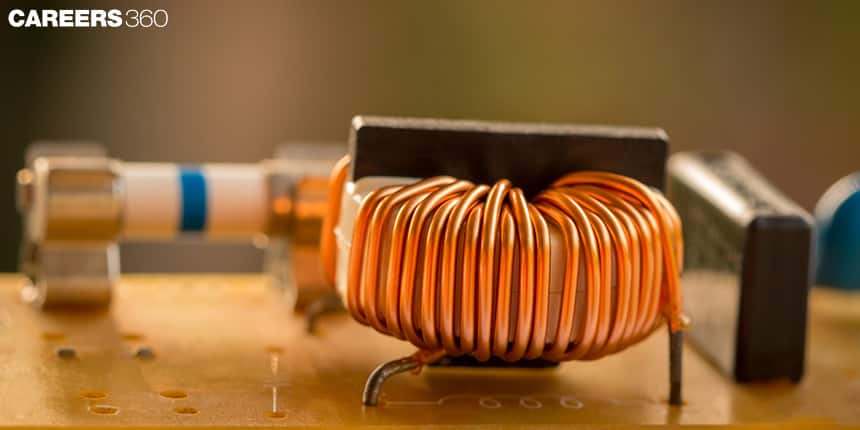###### Synopsis

Electromagnetic induction is very important because it is the base of electrical energy. This principle is used to convert other forms of energy into electrical energy. Also, this is an important unit for National Standard Examination in Physics (NSEP). Because there are one or two questions from the concepts of electromagnetic induction. This article includes formulas, important concepts, and some previous year's questions that can help students to score well in the NSEP.###### Synopsis

Electromagnetic induction is very important because it is the base of electrical energy. This principle is used to convert other forms of energy into electrical energy. Also, this is an important unit for National Standard Examination in Physics (NSEP). Because there are one or two questions from the concepts of electromagnetic induction. This article includes formulas, important concepts, and some previous year's questions that can help students to score well in the NSEP.

Electromagnetic Induction For NSEP - Nowadays we are heavily dependent on electrical energy either stored in batteries or directly supplied by power stations. All the electrical devices like our neckbands, smartwatch, phones, television, Electric cars, metros, electric trains, and many more use electrical energy. There are different forms of energy like hydraulic energy, wind energy, tide energy, Fossil fuel, solar energy, etc. due to their limitations like availability we can not use them properly. However, we convert other forms of energy into electrical energy and that is used everywhere. Do you know how other forms of energy get converted into electrical energy? The branch of physics in which we study how other forms of energy get converted into electrical energy is known as electromagnetic induction.

A turbine converts other forms of energy into electric energy, which works on the principle of electromagnetic induction. When the wings of a turbine rotate using the flow of water or wind or any other energy source then there is a change in the flux and due to this according to Faraday's law, a current is induced. In this way, we are able to convert other forms of energy into electrical energy and that is supplied to every part of the country.

Here in this article we briefly discuss formulas, concepts, and some of the previous year's questions on electromagnetic induction. Interested students can go through the class 12th NCERT chapter on electromagnetic induction to know more about the concepts. They can practice some questions from the chapter to command the concepts.

## Important Concepts In Electromagnetic induction

There are one or two questions based on electromagnetic induction in the National Standard Examination in Physics (NSEP). Let’s discuss concepts of electromagnetic induction.

## Magnetic Flux

The magnetic field can be represented using magnetic field lines and the on-moving charge is proportional to magnetic field lines. Magnetic flux represents the total magnetic lines passing through an area. Magnetic flux is given can be calculated using the given formula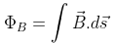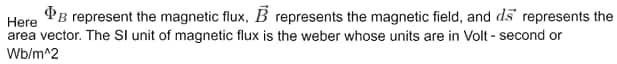Magnetic flux will be zero if the magnetic field is perpendicular to the surface area.

According to Faraday's law, an electromotive force (emf) induces if the magnetic flux through a loop area changes. This emf becomes zero when the magnetic flux stops changing. This emf is equal to the negative rate at which flux is changing.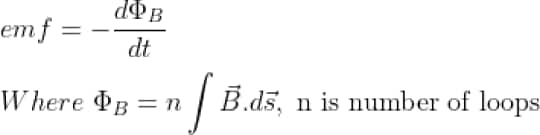If the resistance of the loop is R then the current in the loop is given by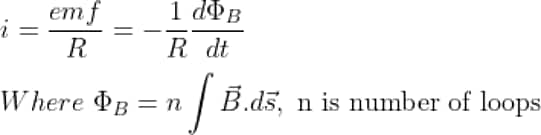There are several ways by which it can change.

1. When magnetic field B changes with time.

2. When the current producing magnetic field changes with a change in the current with time.

3. When the area enclosed by the loop changes

4. When the angle between the magnetic field and the area changes.

5. Any combination of the above changes also causes a change in the flux.

## Lenz’s Law

This law states that the polarity of induced emf or direction of current induced in the loop is such that it opposes the cause by which it is produced.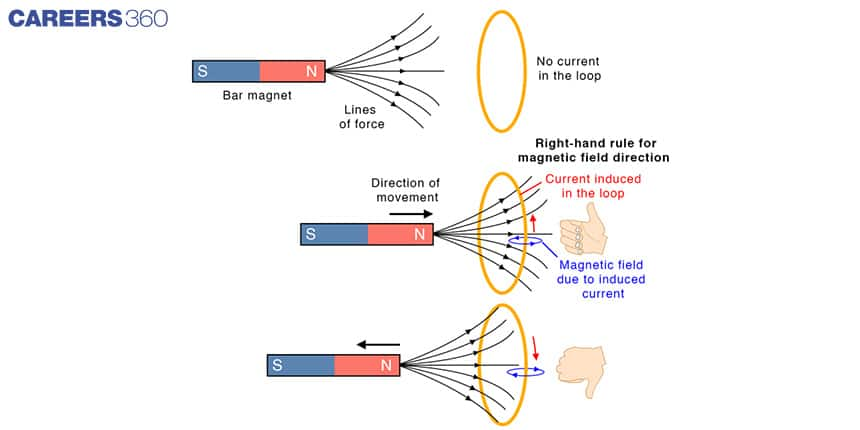## Motional Emf

When a straight conductor wire moves in the magnetic field then electrons inside the conductor experience electric force and they accumulate at the end of the conductor due to that there is a potential difference between the end of the conductor. This conductor wire acts as a battery with the potential difference Blv where B is a magnetic field, L is the length of the conductor and V is the speed of the conductor. This emf is known as motional emf.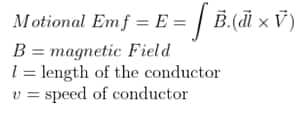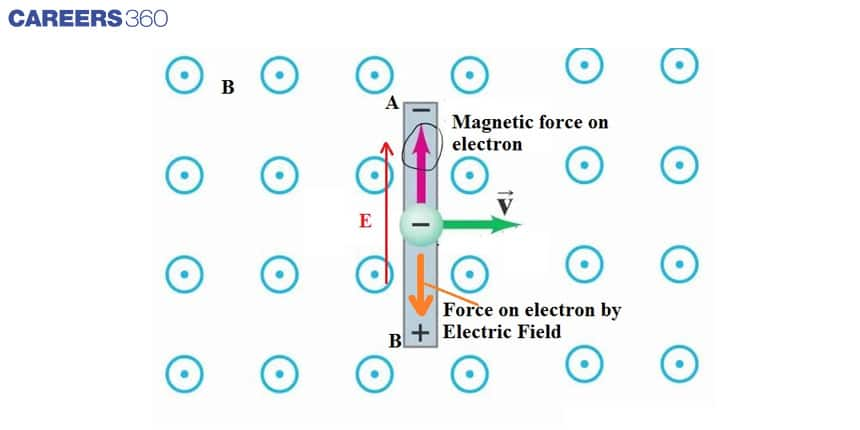## Self-Induction

We have already discussed that capacitors are devices that are used for energy storage and they store energy in the form of an electric field whereas inductors are devices that store energy in the form of a magnetic field. The Inductance of the inductor will depend on the shape of the inductor and the medium in which it is placed.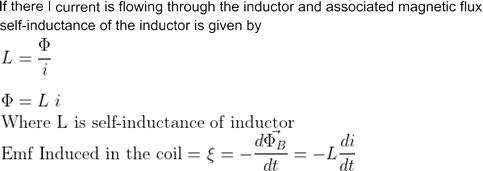the Inductance of the solenoid that has length l, cross-section area A, and number of turns N is given by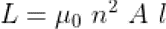## Combination Of Inductor

The concept to find resultant inductance when two or more inductors are connected in series or parallel combinations

1. Series combination: if inductors of inductance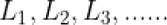are connected in series then the resultant inductance can be calculated as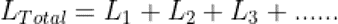2. Parallel combination: if inductorsare connected in parallel combination then the resultant inductance can be calculated as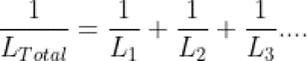## Energy Stored In The Inductor

The Inductor stores energy in form of a magnetic field and this energy is given by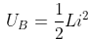## NSEP Previous Year Questions From Electromagnetic induction

Q-1 (NSEP 2018-19)

A thin wire of length 1 m is placed perpendicular to the XY plane. If it is moved with velocity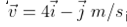in the region of magnetic induction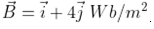. The potential difference developed between the ends of the wire is

1. Zero volt

2. 3 Volt

3. 15 Volt

4. 17 Volt

Q-2 (NSEP 2017-18)

Two identical coils each of self-inductance L, are connected in series and are placed so close to each other that all the flux from one coil links with the other. The total self-inductance of the system is

1. L

2. 2L

3. 3L

4. 4L

Q-3 (NSEP 2017-18)

The physical quantity that has unit volt-second is

1. Energy

2. Electric Flux

3. Magnetic flux

4. Inductance

Q-4 (NSEP 2015-16 )

Two coils wound on the same magnetic core have inductances L1 and L2. When the two coils are connected in series, the effective inductance is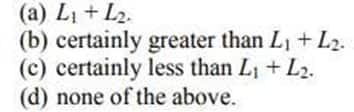Q-5 (NSEP 2015-16)

A coaxial cable consists of two thin cylindrical conducting shells of radii a and b (a<b).

The inductance per unit length of the cable is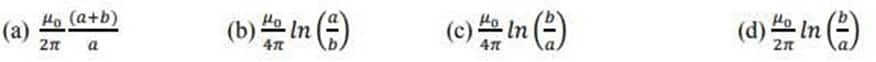## NSEP Study Materials For Electromagnetic induction

• NCERT Class 12 Physics chapter Electromagnetic induction

• NCERT Exemplar problems from Electromagnetic induction

• NSEP previous year papers

• Physical sciences
• Nature
• Physics
• Electromagnetism
• Electricity
• Electrical engineering
• Physical quantities
• Metrology
• Quantity
• Electrodynamics

## Careers360 helping shape your Career for a better tomorrow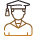#### 250M+

Students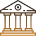#### 30,000+

Colleges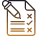#### 500+

Exams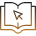E-Books